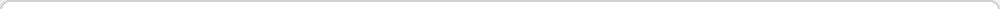# Compound Interest Calculator

Dividend Yield is the "interest rate" you earn from a stock. To get the annual dividend yield first you need to figure out the annual dividend. You can multiply the quarterly dividend by 4, a monthly dividend by 12, or if it is a yearly dividend, just take that. Then plug it, with the share price, into the calculator below.

 Total Yearly Dividend: \$ (the total dividend, such as 0.80) Share Price: \$ (cost of 1 share of stock)